# How to Calculate and Solve for Magnetic Force | Nickzom Calculator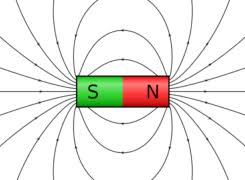The image above represents magnetic force.

To compute the magnetic force of a field, four essential parameters are needed and the parameters are Quantity of Charge (q), Average Velocity of the Charge (v), Magnetic Field Induction or Magnetic Flux Density (B) and Angle between v and B (θ).

The formula for calculating the magnetic force:

F = qVBsinθ

Where;
F = Magnetic Force
q = Quantity of Charge
v = Average Velocity of the Charge
B = Magnetic Field Induction or Magnetic Flux Density
θ = Angle between v and B

Let’s solve an example;
Find the magnetic force of a field when the Quantity of Charge (q) is 11, Average Velocity of the Charge (v) is 20, Magnetic Field Induction or Magnetic Flux Density (B) is 17 and Angle between v and B (θ) is 28°.

This implies that;
q = Quantity of Charge = 11
v = Average Velocity of the Charge = 20
B = Magnetic Field Induction or Magnetic Flux Density = 17
θ = Angle between v and B = 28°

F = qVBsinθ
F = 11 x 20 x 17 x sin28°
F = 11 x 20 x 17 x 0.469
F = 1755.82

Therefore, the magnetic force is 1755.82 Newton (N).

Nickzom Calculator – The Calculator Encyclopedia is capable of calculating the magnetic force.

To get the answer and workings of the magnetic force using the Nickzom Calculator – The Calculator Encyclopedia. First, you need to obtain the app.

You can get this app via any of these means:

To get access to the professional version via web, you need to register and subscribe for NGN 1,500 per annum to have utter access to all functionalities.
You can also try the demo version via https://www.nickzom.org/calculator

Once, you have obtained the calculator encyclopedia app, proceed to the Calculator Map, then click on Magnetic field under the Physics section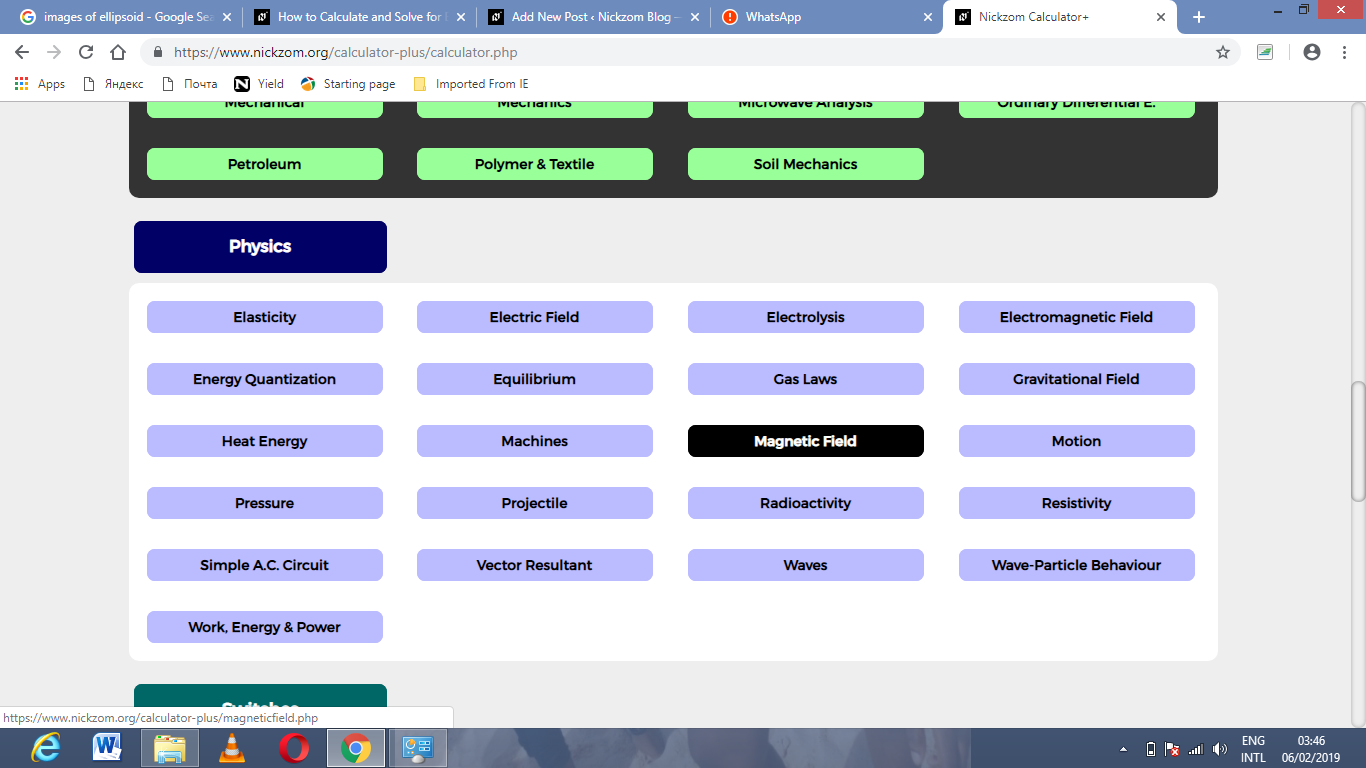Now, Click on Magnetic Force under Magnetic Field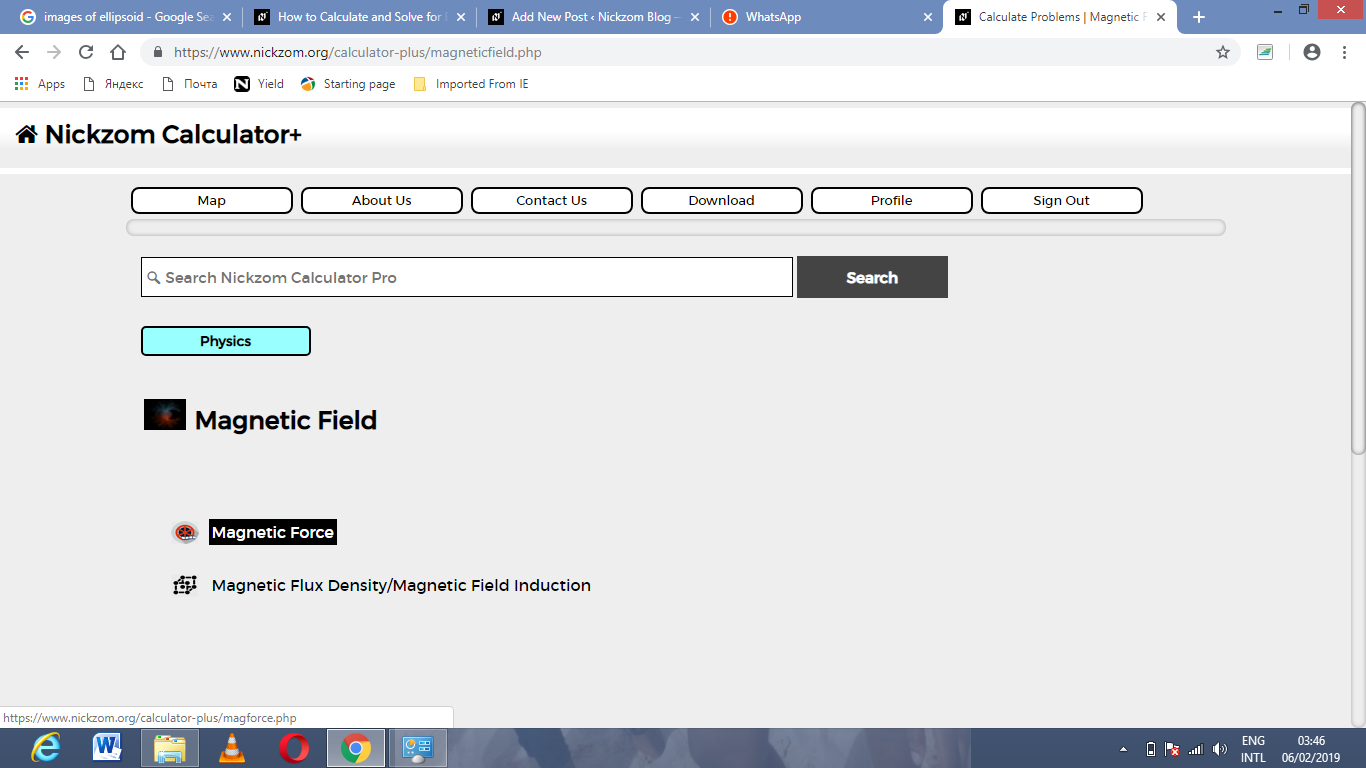The screenshot below displays the page or activity to enter your values, to get the answer for the magnetic force according to the respective parameters which are the Quantity of Charge (q), Average Velocity of the Charge (v), Magnetic Field Induction or Magnetic Flux Density (B) and Angle between v and B (θ).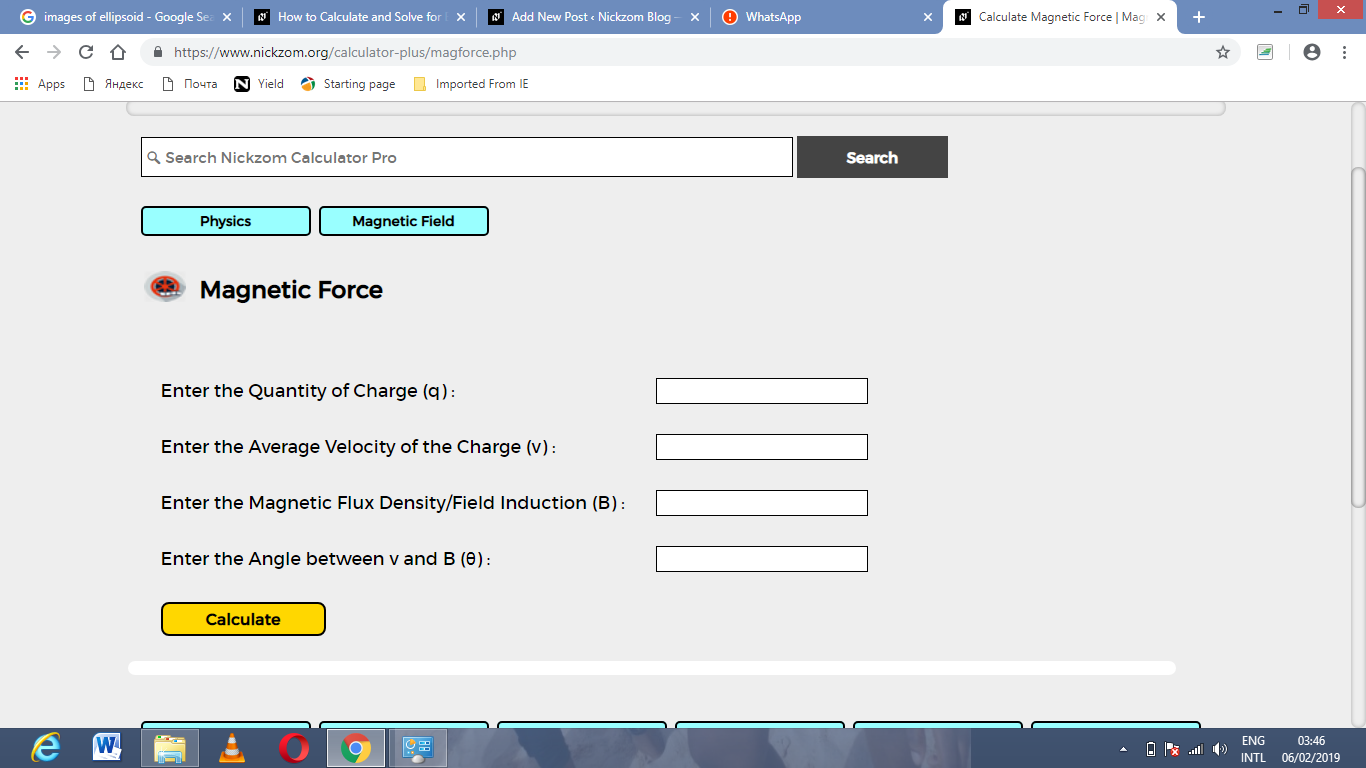Now, enter the values appropriately and accordingly for the parameters as required by the example above where the Quantity of Charge (q) is 11, Average Velocity of the Charge (v) is 20, Magnetic Field Induction or Magnetic Flux Density (B) is 17 and Angle between v and B (θ) is 28°.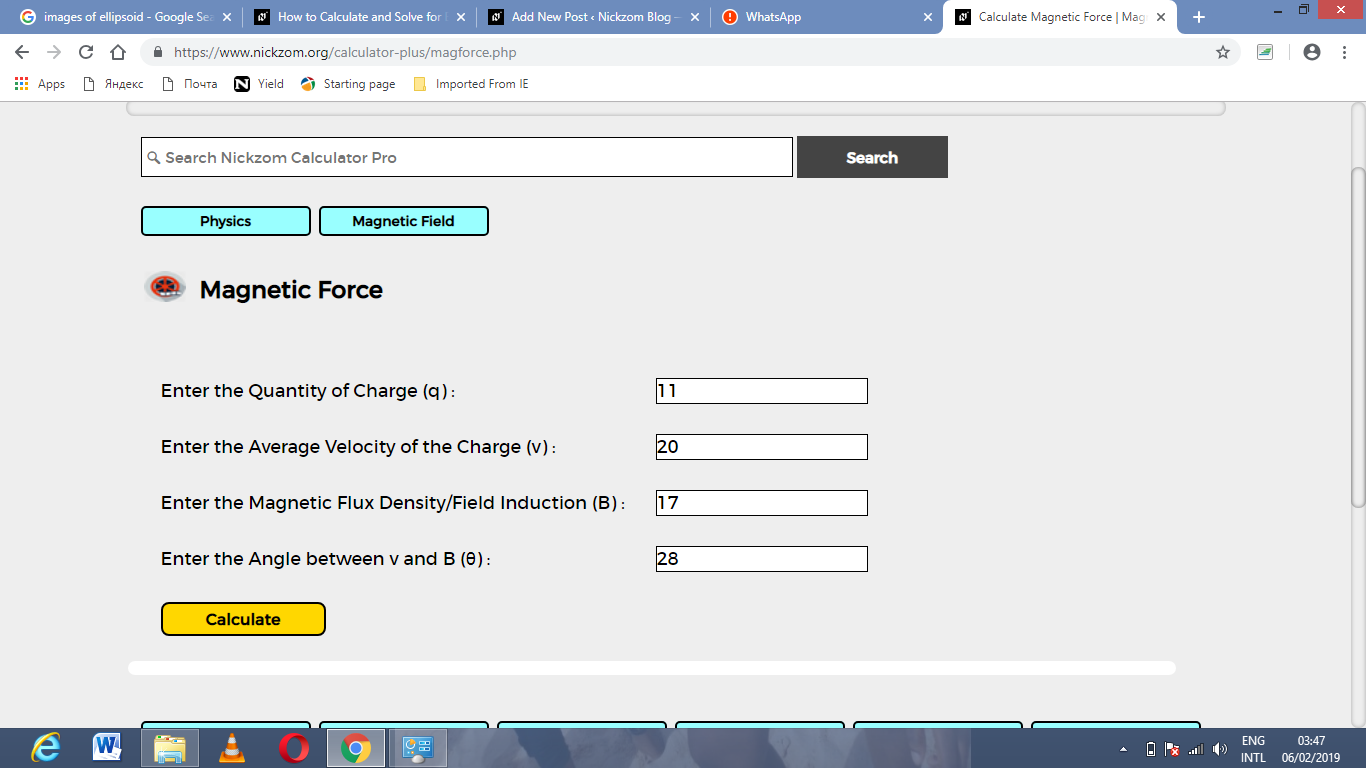Finally, Click on Calculate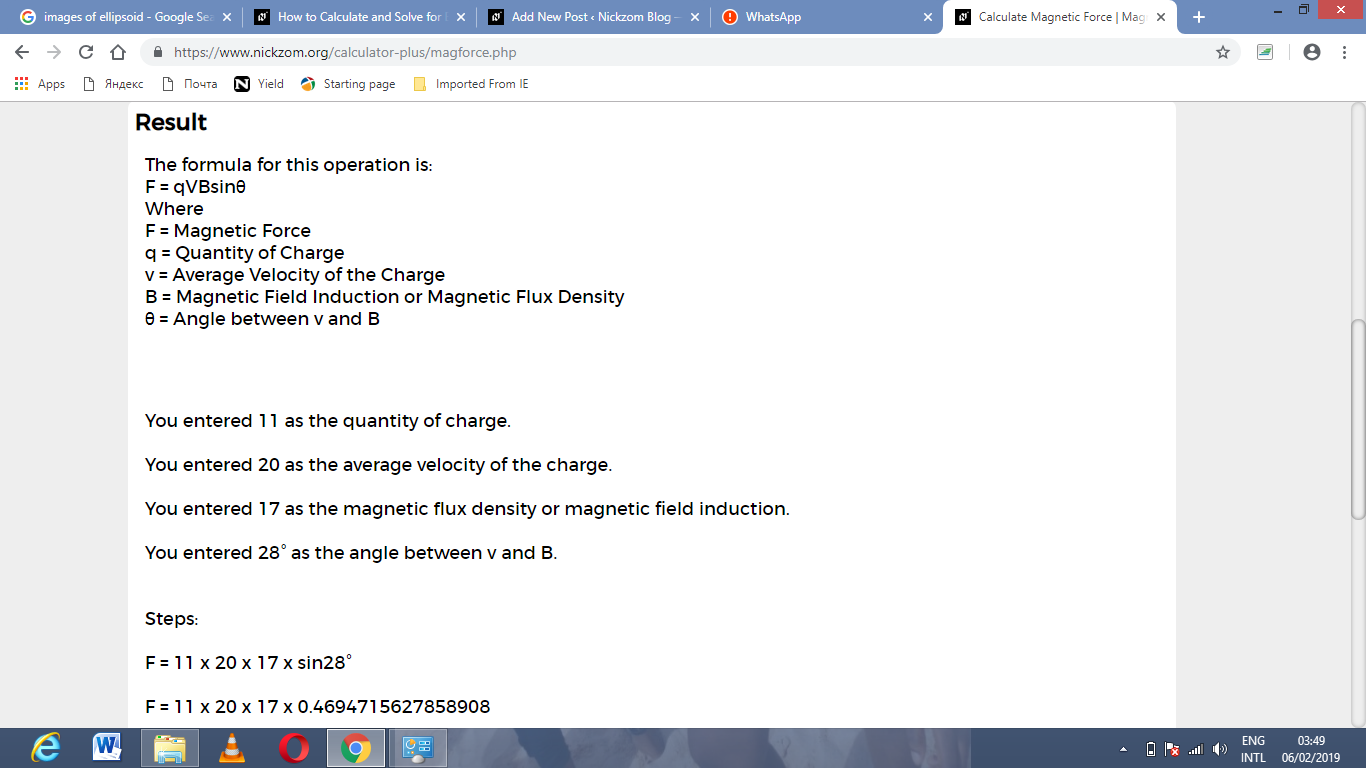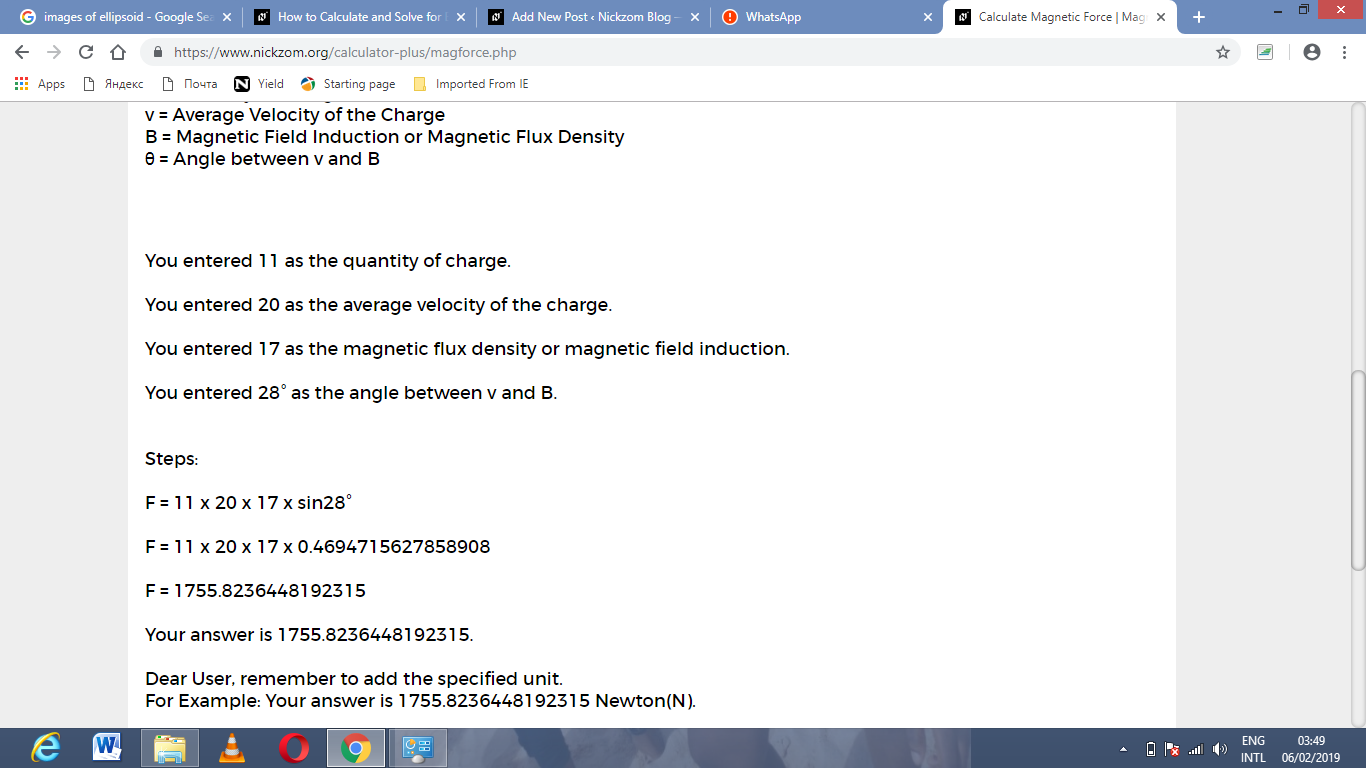As you can see from the screenshot above, Nickzom Calculator – The Calculator Encyclopedia solves for the magnetic force and presents the formula, workings and steps too.